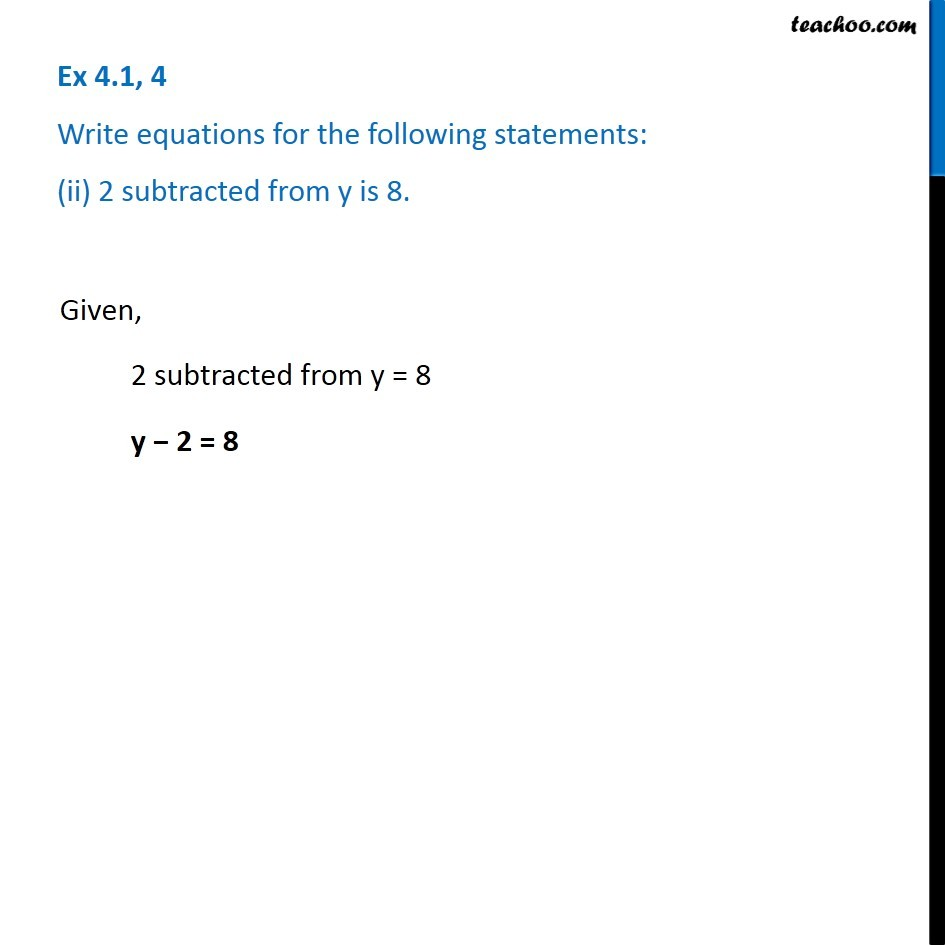Ex 4.1

Chapter 4 Class 7 Simple Equations
Serial order wiseLearn in your speed, with individual attention - Teachoo Maths 1-on-1 Class

### Transcript

Ex 4.1, 4 Write equations for the following statements: (ii) 2 subtracted from y is 8. Given, 2 subtracted from y = 8 y − 2 = 8